# Important Solved Questions of Chapter SolutionsImportant Solved Questions of Chapter Solutions

Que 1. Henry’s Law constant for the solubility of N2 gas in water at 298 K is 1.0 × 105 atm. The mole fraction of N2 in the air is 0.8. Find the number of moles of N2 from air dissolved in 10 moles of eater at 298 K and 5 atm pressure.
Ans 1.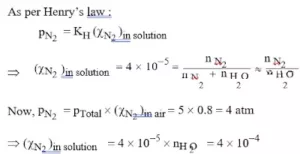Que 2. At a certain temperature, the vapour pressure of pure ether is 646 mm and that of pure acetone is 283 mm. Calculate the mole fraction of each component in the vapour state if the mole fraction of ether in the solution is 0.50.
Ans 2.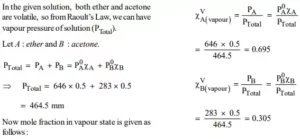Que 3. 4.375 g of a substance when dissolved in 36.0 g of water, lowered its vapour pressure by 0.5 mm at a given temperature. The vapour pressure of water at this temperature is 25.0 mm. Calculate the molecular weight of solute.
Ans 3.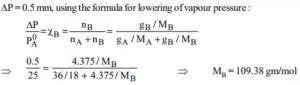Que 4. Assuming ideal behaviour, calculate the vapour pressure of 1.0 molal solution of a non-volatile molecular solute in the water at 500C is 0.122 atm.
Ans 4.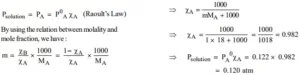Que 5. The vapour pressure of pure water 250C is 23.76 torr. What is the vapour pressure of 100 gm of water to which 100 gm of C6H12O6 (glucose) has been added?
Ans 5.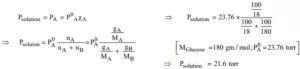Important Solved Questions of Chapter Solutions

Que6.The boiling point of a solution made by dissolving 12.0 gm of glucose in 100 gm of water is 100.340C. Calculate the molecular weight of glucose. Kb for water = 0.520C/m.
Ans 6.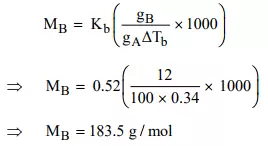Que 7. Blood freezes at 272.44 K and a solution of 3.0 gm of Urea in 250 gm of water freeze at 272.63 K. Calculate the osmotic pressure of blood at 310 K. (Assume density of blood at 310 K to be 1 g/cc).
Ans 7.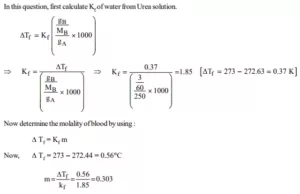Que 8. Calculate the freezing point depression and boiling point elevation of a solution of  10.0 gm of urea (MB  = 60) in 50.0 gm of water at 1 atm. pressure. Kb and Kf for water are 0.520C/m and 1.860C/m respectively.
Ans 8.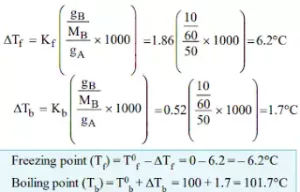Que 9. The boiling point elevation constant for benzene is 2.570C/m. The boiling point of benzene is 80.10C. Determine the boiling point of a solution formed when 5.0 gm of C14H12 is dissolved in 15 gm of benzene.
Ans 9.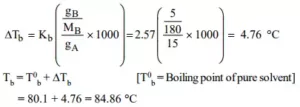Que 10. What mass of sugar, C12H22O11 (MM = 342) must be dissolved in 4.0 kg of H2O to yield a solution that will freeze at –3.72°C. (Take Kf  = 1.86°C/m).
Ans 10.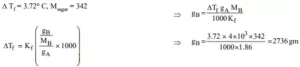Important Solved Questions of Chapter Solutions

Que 11. Acetic acid associates in benzene to form a dimer. 1.65 gm of acetic acid, when dissolved in 100 gm of benzene, raised the B.P. by 0.36 °C. Calculate the Van’t Hoff factor and degree of association of acetic acid.   (Kb for benzene = 2.57 0C/m).
Ans 11.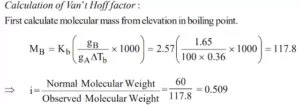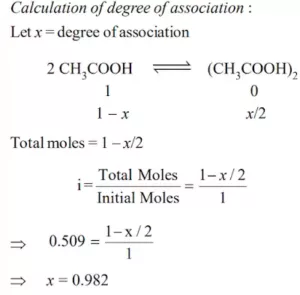Que 12. Find van’t Hoff factor of: a). CH3COOH in H2O,       b). CH3COOH in benzene
Ans 12.   a). When acetic acid dissolves in water, it dissociates into CH3​COO and H+ ions and thus the van’t Hoff factor is greater than 1.
b). Acetic acid dimerizes when dissolved in benzene and thus the van’t Hoff factor is less than 1.

Que 13. A 0.1 M solution of potassium sulphate, K2SO4 is dissolved to the extent of 90%. What would be its osmotic pressure at 27 0C?
Ans 13.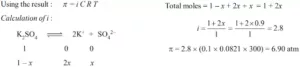Que 14. Why is the vapour pressure of a solution of glucose in water lower than that of water?
Ans 14. When glucose is added to water, the surface has both glucose and water molecules, therefore, escaping tendency of water changing into vapours becomes less and vapour pressure of solution becomes less.

Que 15. What is the molarity of acetic acid containing 6 g of acetic acid per litre of the solution?
Ans 15.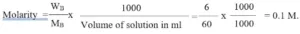Important Solved Questions of Chapter Solutions

Que 16. What is expected van’t Hoff factor for K4 [Fe(CN)6]?
Ans 16.  K4 [Fe(CN)6] → 4K+ + [Fe(CN)6]4- ; van’t Hoff factor (i) = 5.

Que 17. What is anti-freeze?
Ans 17. Anti-freeze is a substance which is added to the solvent to lower its freezing point. It is used in car radiators to lower the freezing point of water, e.g., ethylene glycol.

Que 18. Calculate the volume of 80% (by mass) of H2SO4 (density = 1.80 g/ml) required to prepare 1 litre of 0.2 molar H2SO4, (Relative atomic masses : H = 1, O = 16, S = 32).
Ans 18.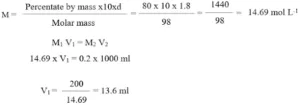Que 19. Give one example each of miscible liquid pairs showing positive and negative deviations from Raoult’s law. Give one reason each for such deviations.
Ans 19. A mixture of ethanol and acetone show a Positive Deviation from Rault’s law.

Explanation: When pure ethanol is added to cyclohexane the molecule of cyclohexane come in between the molecule of ethanol which results in the breaking of hydrogen bond and thus positive deviation.
A mixture of chloroform and acetone shows Negative Deviation.
Explanation: When these are mixed hydrogen bond is formed and negative deviation is shown.

Que 20. a). What is Raoult’s law and what are its applications?
b). Urea forms an ideal solution in water. Determine the vapour pressure of an aqueous solution containing 10% by mass of urea of 40oC. (Vapour pressure of water at 40oC = 55.3 mm of Hg).
Ans 20. a).  Raoult’s law is applicable only to very dilute solutions and according to this law, the vapour pressure of a component at a given temperature is equal to the mole fraction of that component in the solution multiplied by the vapour pressure of that component in the pure state.
b). Mass of urea = 10g
Mass of water = 90g
Moles of urea = 10g/60g mol-1 = 1/6
Moles of water = 90/18 = 5
Mole fraction of water
XA = 5/5+16 = 5/31
Vapour pressure of solution
P = P°A.XA = 55.3×5/31
P = 53.52mm Hg.

Important Solved Questions of Chapter Solutions

Que 21. a).  Draw graph showing depression of freezing point due to non-volatile solute.
b).   Calculate the freezing point of a solution containing 0.520 g of glucose (C6H12O6) dissolved in 80.20 g of water. [For water Kf = 1.86 K kg mol-1.]
Ans 21. (a). A solution will freeze when its vapour pressure equals the vapour pressure of the pure solid solvent. According to Raoult’s law, when a non-volatile solid is added to the solvent its vapour pressure decreases and now it would become equal to that of solid solvent at a lower temperature. Thus, the freezing point of the solvent decreases. The depression in freezing point depends on the number of solute molecules rather than their nature hence, it is a colligative property.

(b).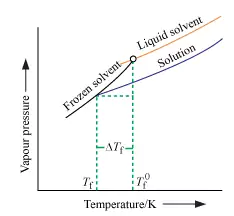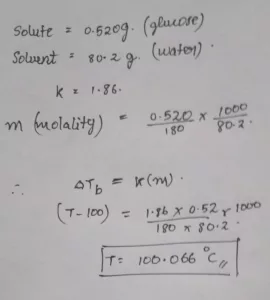Important Solved Questions of Chapter Solutions